International Journal of Discrete Mathematics
Volume 1, Issue 1, December 2016, Pages: 1-4

P. Gayathri1, K. R. Subramanian2

2Department of Computer Applications, Shrimati Indira Gandhi College, Trichy, Tamilnadu, India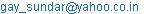(P. Gayathri)

P. Gayathri, K. R. Subramanian. The PI(Padmakar-Ivan) Index of Polyominoes. International Journal of Discrete Mathematics. Vol. 1, No. 1, 2016, pp. 1-4. doi: 10.11648/j.dmath.20160101.11

Received: September 19, 2016; Accepted: November 18, 2016; Published: December 26, 2016

Abstract: The Padmakar – Ivan (PI) index of polyominoes is examined.Efficient calculations of formulas for PI index for the polyominoes are put forward. In chemical graph theory, the PI index is a topological indexof a graph G is defined as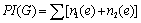, where for edge e = xy, n1(e) is the number of edges of G lying closer to x than y, n2(e) is the number of edges of G lying closer to y than x and summation goes over all edges of G. The edges equidistant from x and y are not considered for the calculation of PI index. In this paper, we calculated the PI index of polyominoes like square Polyomino, L-Polyomino,T-Polyomino, Straight-Polyomino and Skew-Polyomino.

Keywords: Molecular Graph, Polyominoes, Topological Indices, PI (Padmakar-Ivan)Index

Contents

1. Introduction

Chemical Graph Theory is an interdisciplinary science that applies Graph Theory to the study of molecular structures. The molecules or chemical compounds are modeled by an undirected graph - the molecular graph have vertices represent atoms or group of atoms andthe edges represent the chemical bonds between atoms or group of atoms. A topological index is a numerical parameter mathematically derived from the graph structure. It is a graph invariant thus it does not depend on the labeling or pictorial representation of the graph. The topological indices of molecular graphs are widely used for establishing correlations between the structure of a molecular compound and its physico-chemical properties or biological activity for example Pharmacology.A Polyomino system is a finite 2-connected plane graph such that each interior face (or say a cell) is surrounded by a regular square of length one. In other words, it is an edge-connected union of cells in the planar square lattice. For the origin of polyominoes we quote Klarner : "Polyominoes have a long history, going back to the start of the 20th century, but they were popularized in the present era initially by Golomb, i.e. [2, 3], then by Gardner in his Scientific American columns." At the present time they are widely known by mathematicians, physicists, chemists and have been considered in many different applications .

One of the oldest and most thoroughly examined molecular graph-based structural descriptor of organic molecule is the Wiener index or Wiener number [5, 6]. The Wiener index (W) is applicable to acyclic (tree) graphs only. For cyclic compounds a novel molecular-graph-based descriptor, referred to as the Szeged index (Sz) is put forward by Gutman  and co-workers . This is considered as the modification of W to cyclic graph. It is based on distance in the molecular graph but is not of the same type as W. For acyclic systems (trees) Sz and W coincide. Consequently, one of the authorsintroduced yet another index called Padmakar-Ivan (PI) index [9, 10]. The PI index of a graph G is defined as,where for edge e = xy, n1(e) is the number of edges of G lying closer to x than y, n2(e) is the number of edges of G lying closer to y than x and summation goes over all edges of G. The edges equidistant from x and y are not considered for the calculation of PI index. Since the PI index is different for acyclic graphs, several applications of the PI index are reported in the literature [10-13].Many methods for the calculation of PI indices of some systems are reported in [14-17]. Many methods and several applications are reported in the previous literature [18-28] about the graph invariants of Polyominoes. In this paper, we calculated the PI index of polyominoes like Square Polyomino, L-Polyomino, T-Polyomino, Straight Polyomino and Skew Polyomino.

2. Some Important Theorems on PI Index of Polyominoes

Calculation of PI index includes the following procedure:

Step:1

The number of edges and number of squares are calculated according to the segment lengths of the polyominoes using their graph structures.

Step:2

The numbers of parallel edges in are calculated by using the graph structures of polyominoes and results are tabulated.

Step:3

By using the definition of PI index the PI index of the polyominoes are obtained by generalizing the index values based on their segment lengths ‘r’ and the number of edges ‘e’.

Theorem 2.1

Letis a square polyomino where, ‘s’ represents the number of squares, ‘e’ represents the number of edges and ‘r’ represents the number of segments,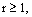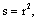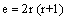for all r, then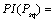Figure 1. Graphs of Square polyominoes with r=1,2,3,4 and 5.

Proof:

From the above graph structures of Square polyominoes, the number of squares in each segment is equal to the square of the segment length, and number edgesare calculated and then the number of parallel edges and their corresponding number of sets for r equal to the 1,2,3 and 4 are obtained and tabulated as given below.

Table 1. Calculation of PI index for square polyomino for r=1,2,3 and 4.

 In square Polyomino No. of. parallel edges No. of. sets PIIndex For r=1,e=4,s=1 2 2 4(e-2) For r=2,e=12,s=4 3 4 12(e-3) For r=3,e=24,s=9 4 6 24(e-4) For r=4,e=40,s=16 5 8 40(e-5)

PI Index of Square Polyomino

=No. of edges (No. of edges-No. of segments)=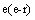=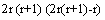since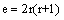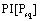(1)

Theorem 2

Let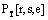is a T- polyomino where, ‘s’ represents the number of squares, ‘e’ represents the number of edges and ‘r’ represents the number of segments,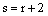,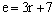for all r, then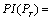Figure 2. Graphs of T-Polyominoes with r=2,3,4,5 and 6.

Proof:

From the above graph structures of T-Polyominoes, the number of squares in each segment is equal to two more than their corresponding segment length, and number edgesare calculated and then the number of parallel edges and their corresponding number of sets for r equal to the 2,3,4 and 5are obtained and tabulated as given below.

Table 2. Calculation of PI index for T-Polyomino for r=2,3,4 and 5.

 In T- Polyomino No. of. parallel edges No. of. Sets PIIndex For r = 2, e=13, s=4 4 1 4(e-4) 3 1 3(e-3) 2 3 6(e-2) For r = 3, e=16, s=5 4 1 4(e-4) 4 1 4(e-4) 2 4 8(e-2) For r = 4, e=19, s=6 4 1 4(e-4) 5 1 5(e-3) 2 5 10(e-2) For r = 5, e=21, s=7 4 1 4(e-4) 6 1 6(e-3) 2 6 12(e-2)

PI Index of T- Polyomino

=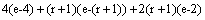=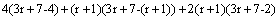since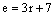(2)

Theorem 3

Let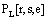is a L- polyomino where, ‘s’ represents the number of squares, ‘e’ represents the number of edges and ‘r’ represents the number of segments,, with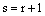,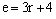for any r, then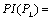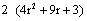Figure 3. Graphs of L-Polyominoes with r=2,3,4,5 and 6.

Proof:

From the above graph structures of L-Polyominoes, the number of squares in each segment is equal to one more than their corresponding segment length, and number edgesare calculated and then the number of parallel edges and their corresponding number of sets for r equal to the 2,3,4 and 5 are obtained and tabulated as given below.

Table 3. Calculation of PI index for square polyomino for r=1,2,3 and 4.

 In L- Polyomino No. of. parallel edges No. of. Sets PIIndex For r = 2, e =10, s = 3 3 1 3(e-3) 3 1 3(e-3) 2 2 4(e-2) For r = 3,e = 13, s = 4 3 1 3(e-3) 4 1 4(e-4) 2 3 6(e-2) For r = 4,e =16, s = 5 3 1 3(e-3) 5 1 5(e-5) 2 4 8(e-2) For r = 5, e=19, s = 6 3 1 3(e-3) 6 1 6(e-6) 2 5 10(e-2)

PI Index of L- Polyomino

=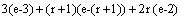=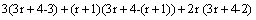since=(3)

Theorem 4

Let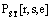is a straight polyomino where, ‘s’ represents the number of squares, ‘e’ represents the number of edges and ‘r’ represents the number of segments,, with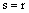,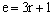for any r, then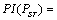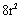Figure 4. Graphs of Straight Polyominoes with r=1,2,3,4,5 and 6.

Proof:

From the above graph structures of Straight Polyominoes, the number of squares in each segment is equal totheir corresponding segment length, and number edgesare calculated and then the number of parallel edges and their corresponding number of sets for r equal to the 1,2,3,4 and 5 are obtained and tabulated as given below.

Table 4. Calculation of PI index for straight polyomino for r=1,2,3,4 and 5.

 InStraightPolyomino No. of. parallel edges No. of. sets PIIndex For r=1, e=4, s=1 2 2 4(e-2) For r=2, e=7, s=2 2 2 4(e-2) 3 1 3(e-3) For r=3, e=10, s=3 2 3 6(e-2) 4 1 4(e-4) For r=4, e=13, s=4 2 4 8(e-2) 5 1 5(e-5) For r=5, e=16, s=5 2 5 10(e-2) 6 1 6(e-6)

PI Index of Straight- Polyomino

=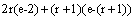=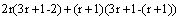since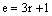=(4)

Theorem 5

Let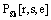is a skew polyomino where, ‘s’ represents the number of squares, ‘e’ represents the number of edges and ‘r’ represents the number of segments,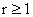, with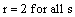,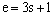forr = 2, then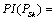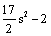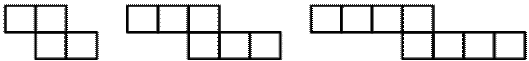Figure 5. Graphs of Skew Polyominoes with r=2 for s=4,6 and 8.

Proof:

From the above graph structures of Skewt Polyominoes, the number of segmentsis two for all graphs withdifferent number of squares and number of edgesare calculated and then the number of parallel edges and their corresponding number of sets for r equal to 2 and s=2,6,8 and 10 are obtained and tabulated as given below.

Table 5. Calculation of PI index for square polyomino for r=1,2,3 and 4.

 In Skew - Polyomino No. of. parallel edges No. of. sets PIIndex For r=2, e=13, s=4 3 1 3(e-3) 3 2 6(e-3) 2 2 4(e-2) For r=2, e=19, s=6 3 1 3(e-3) 4 2 8(e-4) 2 4 8(e-2) For r=2, e=25, s=8 3 1 3(e-3) 5 2 10(e-5) 2 6 12(e-2) For r=2, e=31, s=10 3 1 3(e-3) 6 2 12(e-6) 2 8 16(e-2)

PI Index of Skew- Polyomino

==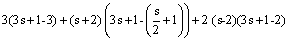since=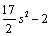(5)

3. Conclusion

A topological index is a molecular descriptor that is calculated based on the molecular graph, of a chemical compound. Several scientists are involved in searching for new molecular descriptors able to catch new aspects of the molecular structure. This kind of research involves creativity and imagination together with solid theoretical basis allowing to obtain numbers with some structural chemical meaning.These type of indices are playing a significant role in theoretical chemistry specially in QPSR/QSAR research that is molecular connectivity in chemistry and Drug research. In this approach, the PI indices found as closed formula for the family of graphs and also it will be very useful for the chemist to calculate the indices by using the newly arrived functional values.

References

1. D. A. Klarner, Polyominoes, in: J. E. Goodman, J. O’Rourke (Eds.), Handbook of Discrete and Computational Geometry, CRC Press LLC,1997, pp. 225–242 (Chapter 12).
2. P. E. John, P. V. Khadikar, J. Singh, A method of computing the PI index of benzenoid hydrocarbons using orthogonal cuts, J. Math. Chem. 42(1) (2007) 37–45.
3. S. W. Golomb, Polyominoes, Charles Scribner’s Sons, 1965.
4. S. W. Golomb, Checker boards and polyominoes, Amer. Math. Monthly 61 (1954) 675–682.
5. H. Wiener, Structural determination of paraffin boiling points, J. Amer. Chem. Soc. 69 (1947) 17–20.
6. R. Todeschini, V. Consodni, Handbook of Molecular Descriptors, Willey-VCH, Weinheim, 2000.
7. I. Gutman, A formula for the Wiener number of trees and its extension to graphs containing cycles, Graph Theory Notes New York 27 (1994)9–15.
8. P. V. Khadikar, N. V. Deshpande, P. P. Kale, A. Dobrynin, I. Gutman, G. J. Domotor, The Szeged index and an analogy with the Wiener index, J. Chem. Inform. Comput. Sci. 35 (1995) 547–550.
9. P. V. Khadikar, P. P. Kale, N. V. Deshpande, S. Karmarkar, V. K. Agrawal, Novel PI indices of hexagonal chains, J. Math. Chem. 29 (2001)143–150.
10. P. V. Khadikar, S. Karmarkar, V. K. Agrawal, A novel PI index and its applications to QSRP/QSAR studies, J. Chem. Inf. Comput. Sci. 41 (4)(2001) 934–949.
11. P. V. Khadikar, P. P. Kale, N. V. Deshpande, S. Karmarkar, V. K. Agrawal, Novel PI indices of hexagonal chains, J. Math. Chem. 29 (2001)143–150.
12. P. V. Khadikar, A. Phadnis, A. Shrivastava, QSAR study on toxicity to aqueous organism using PI index, Bioorg. Med. Chem. 10 (2002)1181–1188.
13. H. Deng, Extremal catacondensed hexagonal systems with respect to the PI index, MATCHCommun. Math. Comput. Chem. 55 (2) (2006)453–460.
14. H. Deng, S. Chen, The PI index of pericondensed benzenoid graphs, J. Math. Chem. (2006), doi:10.1007/s10910-006-9175-9.
15. H. Deng, The PI index of TUVC6[2p, q], MATCH Commun. Math. Comput. Chem. 55 (2) (2006) 461–476.
16. H. Deng, S. Chen, J. Zhang, The PI index of Phenylenes, J. Math. Chem. 41 (1) (2007) 63–69.
17. L. Alonso, R. Cerf, The three dimensional polyominoes of minimal area, Electron. J. Combin. 3 (1996) 39p.
18. I. Gutman, N. Trinajstic, Graph theory and molecular orbitals, totalπ-electron energy of alternant hydrocarbons, Chem. Phys. Lett. 17 (1972) 535–538.
19. I. Gutman, K. C. Das, The first Zagreb index 30 years after, MATCH Commun. Math. Comput. Chem. 50 (2004) 83–92.
20. S. Nikolic, G. Kovačevic, A. Miličevic, N. Trinajstic, The Zagreb indices 30 years after, Croat. Chem. Acta 76 (2003) 113–124.
21. B. Zhou, I. Gutman, Relations between Wiener, hyper-Wiener and Zagreb indices, Chem. Phys. Lett. 394 (2004) 93–95.
22. B. Zhou, Zagreb indices, MATCH Commun. Math. Comput. Chem. 52 (2004) 113–118.
23. B. Zhou, I. Gutman, Further properties of Zagreb indices, MATCH Commun. Math. Comput. Chem. 54 (2005) 233–239.
24. D. A. Klarner, Polyominoes, in: J.E. Goodman, J. O’Rourke (Eds.), Handbook of Discrete and Computational Geometry, CRC Press LLC, 1997, pp. 225–242 (Chapter 12).
25. Y. Zeng, F. Zhang, Extremal polyomino chains on k-matchings and k-independent sets, J. Math. Chem. 42 (2) (2007) 125–140.
26. L. Xu, S. Chen, The PI index of polyomino chains, Appl. Math. Lett. 21 (2008) 1101–1104.
27. J. Yang, F. Xia, S. Chen, On the Randic index of of polyomino chains, Appl. Math. Sci. 5 (5) (2011) 255–260.
28. J. Yang, F. Xia, S. Chen, On sum-connectivity index of polyomino chains, Appl. Math. Sci. 5 (6) (2011) 267–271.

 Contents 1. 2. 3.
Article ToolsAbstractPDF(254K)